## Candice is preparing for her final exam in Statistics. She knows she needs an 62 out of 100 to earn an A overall in the course. Her instruct

Question

Candice is preparing for her final exam in Statistics. She knows she needs an 62 out of 100 to earn an A overall in the course. Her instructor provided the following information to the students. On the final, 200 students have taken it with a mean score of 54 and a standard deviation of 6. Assume the distribution of scores is bell-shaped. Calculate to see if a score of 62 is within one standard deviation of the mean.

in progress 0
2 months 2021-07-22T18:43:33+00:00 1 Answers 3 views 0

A score of 62 is not within one standard deviation of the mean.

Step-by-step explanation:

When the distribution is normal, we use the z-score formula.

In a set with mean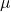and standard deviation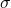, the zscore of a measure X is given by: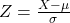The Z-score measures how many standard deviations the measure is from the mean. After finding the Z-score, we look at the z-score table and find the p-value associated with this z-score. This p-value is the probability that the value of the measure is smaller than X, that is, the percentile of X. Subtracting 1 by the pvalue, we get the probability that the value of the measure is greater than X.

Mean score of 54 and a standard deviation of 6.

This means that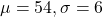Calculate to see if a score of 62 is within one standard deviation of the mean.

Is Z between -1 and 1 when X = 62?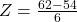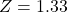So a score of 62 is not within one standard deviation of the mean.